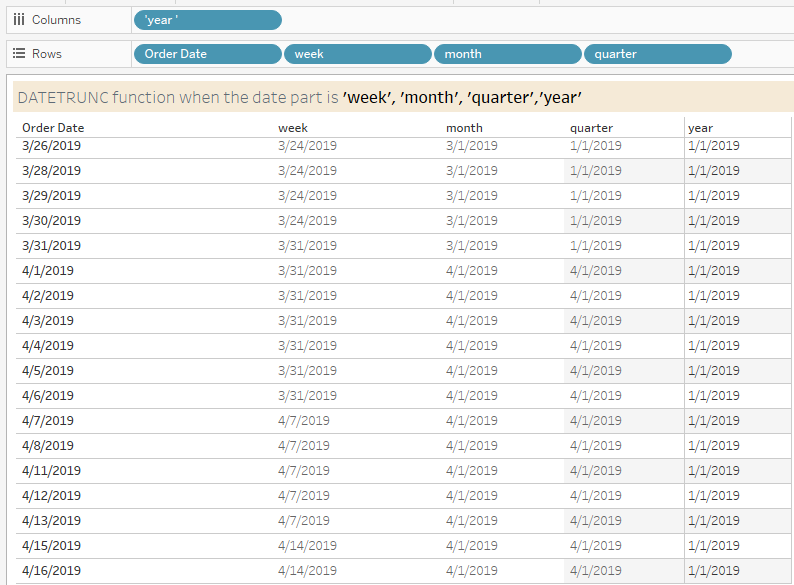top of page
Search
• Bernard K

# DATETRUNC () Function in Tableau### Introduction

DATETRUNC function is used to truncate a specific date into the accuracy specified by the date part.

Syntax: DATETRUNC (date part, date, [start-of-week])

E.g., DATETRUNC (‘quarter’, [Order Date], ‘Sunday’) -> which truncates the date field to the first day of each quarter, with Sunday being taken as the start of the week.

DATETRUNC (‘month’, [Order Date]) -> This function truncates the date field to the first day of each month, with the start of the week assuming the day configured in the data source.

You can edit/configure your data source week start by,

Right-clicking on the data source -> Date Properties…(Note: If the start of the week is omitted in the DATETRUNC calculation, the week start day is determined by the start day configured in the data source).

### Variations of the DATETRUNC function

Below see the results of the DATETRUNC function.

DATETRUNC (date part, Order Date) – when date part is applied as week, month, quarter, and year respectively.(All dates are truncated to the first day of each date part)

### Application of the DATETRUNC function

One of the ways in which I apply the DATETRUNC function is when creating a stepped line chart. For example, I can create a stepped line chart visualizing Sales by month…. By first calculating sales generated in each month by simply combining a FIXED LOD & a DATETRUNC function.(This calculation truncates the Order Date to the first day of each month, then evaluates the sales generated in each of the month).

To build the view (stepped line chart)

• Right-drag Order Date to the column shelf à Order Date (Continuous)

• Add the calculation ‘Sales by month’ to the row shelf(Which creates the stepped line chart above by plotting the monthly sales value on the first day of the month, while repeating the same value for the remainder days of the month – forming a horizontal gap/line between one month to the next)

### Conclusion

If this post was helpful and you would like to receive more of these Tableau tips and tricks, kindly subscribe to our mailing list below.

If you like the work we do and would like to work with us, drop us an email on our Contacts page and we’ll reach out!